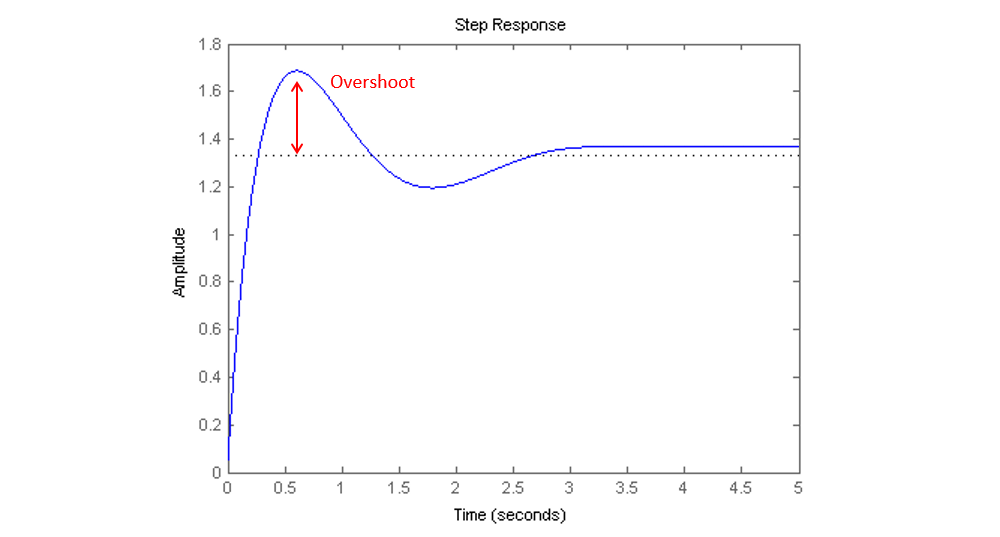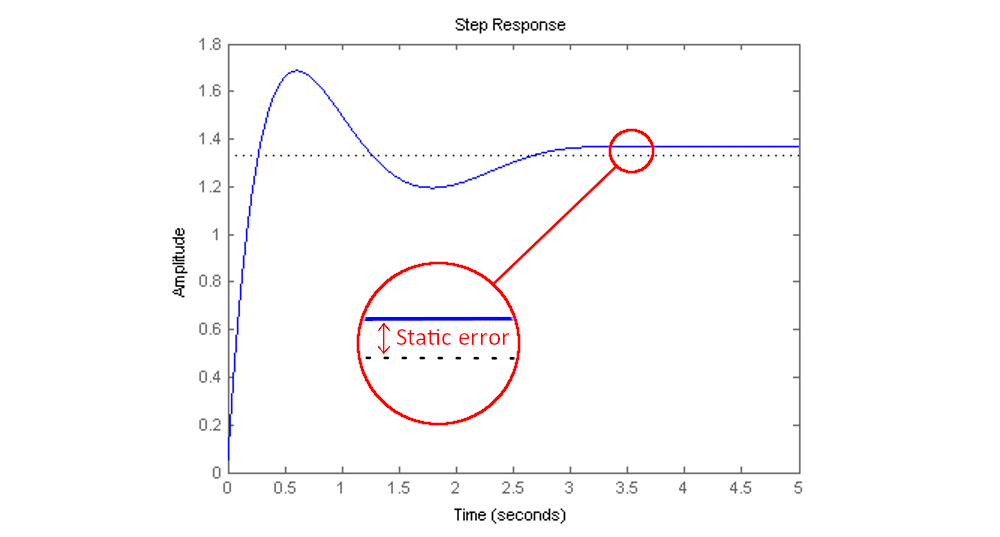# PID: the P as in Proportional

This post is the follow up of the introduction post to PID control for DC motors. They can be read by virtually anyone who can read English, but I highly suggest you to read the introduction post first, as well as the basics about motors listed at the end of this post.

In the previous post I introduced to you the PID control. In this post and the next ones we will discuss about how it works. This is how I divided the whole thing: one letter for one post. As PID has 3 letters, I let you do the maths (no calculator authorized).

So, welcome to the _P-_post.

As you can remember from my previous writing, a PID is a coded feedback loop control— sometimes it’s hardware, but most of the time it’s software —, working into a system including at least:

• a powered actuator (eg. a DC motor),
• a sensor (eg. a speed sensor), and,
• the associated command (most of the time an electronic board with a microprocessor).

Basically, a PID is a tool used for politely but firmly asking a machine to move or react exactly the way you want, while adapting to its changing environment.

Here is some must-known free vocabulary you will need to go on reading:

• Set-point: defined value to be reached by the actuator.
• Process variable: real actuator’s value, retrieved by the sensor in real time.
• Error: difference between set-point and process variable.
• Steady state: when the system stops oscillating and finds its full balance.

The controlled actuator is required to reach a set-point (speed, position, temperature, etc.). The PID uses the process variable and the set-point as a feedback, in the form of an error, to correct the command sent to the actuator so that it can be as precise and fast as possible to get to the set-point.

This is the formula behind the explanation:This is the complete form of a PID, though you can use a P, a PI or a PD if you consider that it’s enough for your kick-ass robot application.

Here, the letter P stands for proportional. We then chose a simple coefficient KP. It is a value that is multiplied by the error, and that’s why it’s called like that:

The P command is proportional to the error. That means the bigger the error, the bigger the command to correct it.

And that would be the only thing you must remember for the proportional part. In a mathematical form, it looks like that:And, if I only want a P command instead of a PID command:Here are the simplified steps of the action of the proportional. I chose, what a surprise, the example of a DC motor which directly drives a propeller — i.e. no reduction involved. In this example you want to control the speed of the propeller:

• The set-point, a rotational speed value you have chosen, is sent to the motor.
• The motor receives the order to reach the set-point speed, but as it begins motionless, the difference between the set-point and the process variable, the error, is high.
• The error value is then multiplied by the KP value you put into the command and a new order is sent to the motor.
• The motor receives the new order and accelerates, so the error decreases.
• The error being lower, the command decreases as well…
• …and so on, until the set-point’s speed is reached by the motor, at steady state.
• As soon as the speed decreases, the error increases to correct the speed, in a set of small variations.

Note: the speed value reached by the motor and read by the sensor (process variable) can sometimes overcome the set-point’s value, resulting in a change of sign of the error. This results in an overshoot.At this point, it’s important to note that this example is only about the speed control of a DC motor with a fixed output’s weight. If the motor’s job is about lifting and positioning various weights (which is not a speed control but a position control), a P command won’t be enough anymore. We will see that on the next posts.

Getting back to the subject, what the KP value really does on the system?

## The effects of P

• It has an effect on the speed response: if KP is too low, the system is responding slowly and may not reach the set-point until quite a long time. Increasing KP will speed up the system’s response.
• But: if KP is too high, the system is responding quickly, but the first overshoot may be too big, resulting in a second overshoot the other way down, and so on. The system could become unstable.

Viewed under this angle, the KP value can be considered — even if it’s a simplified consideration— as a direct strength value, because it gives stiffness to the command.

Also, people usually use to view proportional command as a direct action in the present, like the weight of an object acting on it. If I talk of present, maybe I will tell you some things about past and future later… We will get to that on the next few posts.

## The static error of P

There is a drawback of using only proportional: in the previous figure, you may have notice an offset at steady state. But while using the proportional part only of a PID, i.e. I and D are null, a constant difference between the set-point and the process variable appears at steady state. It’s called static error or steady state error.

This static error exists because the more loops, the closer the system is to the set-point, and the smaller the error is. And when the error is closing to zero, there is not enough strength for the system to actually reach the set-point.Spoiler alert, an integrator will resolve this static error in the next post.

The goal now is to tune your KP value in order to have the correct desired response of your system (which is your motor, in this example). It can be done manually, which consists in trying different KP values in the command to find the one that makes your motor respond like you want.

Or you can write a kick-ass program so that it does that on its own while you go take a nap.

Although a simple P command can make the job according to what you want to do with your system, keep in mind that sometimes a PI, a PD or a PID are required for the different robotic applications. These are the subjects of the next posts.

## To summarize

• A P command is a simple proportional command used as a control loop feedback to control a system — in this post, the speed of DC motor as an example.
• It calculates the error as the difference between set-point and process variable, and multiplies it by a factor KP to lower the error in the next loop, so that the system can reach the set-point.
• The proportional command adds responsiveness to the system and makes it faster, but the factor KP must be set so that the system responds quickly and with stability, avoiding too big overshoots.
• A proportional command used alone implies a static error at steady state.
• As a result, a P command alone is sometimes not enough to properly control a system. For example, a varying weight at the output of a motor may require a PI, a PD, or a PID command.

Follow the guide and read the following post where we will talk about Integral, the I in PID.

Get Started with Luos

Tags: